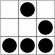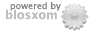"In het verleden behaalde resultaten bieden geen garanties voor de toekomst"

These are the ramblings of Matthijs Kooijman, concerning the software he hacks on, hobbies he has and occasionally his personal life.

Questions? Praise? Blame? Feel free to contact me.

My old blog (pre-2006) is also still available.

Sun Mon Tue Wed Thu Fri Sat
9

Tag Cloud&(With plugins: config, extensionless, hide, tagging, Markdown, macros, breadcrumbs, calendar, directorybrowse, entries_index, feedback, flavourdir, include, interpolate_fancy, listplugins, menu, pagetype, preview, seemore, storynum, storytitle, writeback_recent, moreentries)
Valid XHTML 1.0 Strict & CSS
/ Blog / Blog
Using MathJax math expressions in Markdown

For this blog, I wanted to include some nicely-formatted formulas. An easy way to do so, is to use MathJax, a javascript-based math processor where you can write formulas using (among others) the often-used Tex math syntax.

However, I use Markdown to write my blogposts and including formulas directly in the text can be problematic because Markdown might interpret part of my math expressions as Markdown and transform them before MathJax has had a chance to look at them. In this post, I present a customized MathJax configuration that solves this problem in a reasonable elegant way.

An obvious solution is to put the match expression in Markdown code blocks (or inline code using backticks), but by default MathJax does not process these. MathJax can be reconfigured to also typeset the contents of <code> and/or <pre> elements, but since actual code will likely contain parts that look like math expressions, this will likely cause your code to be messed up.

This problem was described in more detail by Yihui Xie in a blogpost, along with a solution that preprocesses the DOM to look for <code> tags that start and end with an math expression start and end marker, and if so strip away the <code> tag so that MathJax will process the expression later. Additionally, he translates any expression contained in single dollar signs (which is the traditional Tex way to specify inline math) to an expression wrapped in $$ and $$, which is the only way to specify inline math in MathJax (single dollars are disabled since they would be too likely to cause false positives).

# Improved solution

I considered using his solution, but it explicitly excludes code blocks (which are rendered as a <pre> tag containing a <code> tag in Markdown), and I wanted to use code blocks for centered math expressions (since that looks better without the backticks in my Markdown source). Also, I did not really like that the script modifies the DOM and has a bunch of regexes that hardcode what a math formula looks like.

So I made an alternative implementation that configures MathJax to behave as intended. This is done by overriding the normal automatic typesetting in the pageReady function and instead explicitly typesetting all code tags that contain exactly one math expression. Unlike the solution by Yihui Xie, this:

• Lets MathJax decide what is and is not a math expression. This means that it will also work for other MathJax input plugins, or with non-standard tex input configuration.
• Only typesets string-based input types (e.g. TeX but not MathML), since I did not try to figure out how the node-based inputs work.
• Does not typeset anything except for these selected <code> elements (e.g. no formulas in normal text), because the default typesetting is replaced.
• Also typesets formulas in <code> elements inside <pre> elements (but this can be easily changed using the parent tag check from Yihui Xie's code).
• Enables typesetting of single-dollar inline math expressions by changing MathJax config instead of modifying the delimeters in the DOM. This will not produce false positive matches in regular text, since typesetting is only done on selected code tags anyway.
• Runs from the MathJax pageReady event, so the script does not have to be at the end of the HTML page.

You can find the MathJax configuration for this inline at the end of this post. To use it, just put the script tag in your HTML before the MathJax script tag (or see the MathJax docs for other ways).

# Examples

To use it, just use the normal tex math syntax (using single or double $ signs) inside a code block (using backticks or an indented block) in any combination. Typically, you would use single $ delimeters together with backticks for inline math. You'll have to make sure that the code block contains exactly a single MathJax expression (and maybe some whitespace), but nothing else. E.g. this Markdown:

Formulas *can* be inline: $z = x + y$.

Renders as: Formulas can be inline: $z = x + y$.

The double $$ delimeter produces a centered math expression. This works within backticks (like Yihui shows) but I think it looks better in the Markdown if you use an indented block (which Yihui's code does not support). So for example this Markdown (note the indent): $$a^2 + b^2 = c^2$$ Renders as: $$a^2 + b^2 = c^2$$ Then you can also use more complex, multiline expressions. This indented block of Markdown: $$
\begin{vmatrix}
a & b\\
c & d
\end{vmatrix}
$$ Renders as: $$
\begin{vmatrix}
a & b\\
c & d
\end{vmatrix}
$$ Note that to get Markdown to display the above example blocks, i.e. code blocks that start and with $$, without having MathJax process them, I used some literal HTML in my Markdown source. For example, in my blog's markdown source, the first block above literall looks like this:

<pre><code><span></span>    $$a^2 + b^2 = c^2$$</code></pre>


Markdown leaves the HTML tags alone, and the empty span ensures that the script below does not process the contents of the code block (since it only processes code blocks where the full contents of the block are valid MathJax code).

# The code

So, here is the script that I am now using on this blog:

<script type="text/javascript">
MathJax = {
options: {
// Remove <code> tags from the blacklist. Even though we pass an
// explicit list of elements to process, this blacklist is still
// applied.
skipHtmlTags: { '[-]': ['code'] },
},
tex: {
// By default, only \( is enabled for inline math, to prevent false
// positives. Since we already only process code blocks that contain
// exactly one math expression and nothing else, it is also fine to
// use the nicer $...$ construct for inline math.
inlineMath: { '[+]': [['$', '$']] },
},
startup: {
// This is called on page ready and replaces the default MathJax
// "typeset entire document" code.
var codes = document.getElementsByTagName('code');
var to_typeset = [];
for (var i = 0; i < codes.length; i++) {
var code = codes[i];
// Only allow code elements that just contain text, no subelements
if (code.childElementCount === 0) {
var text = code.textContent.trim();
inputs = MathJax.startup.getInputJax();
// For each of the configured input processors, see if the
// text contains a single math expression that encompasses the
// entire text. If so, typeset it.
for (var j = 0; j < inputs.length; j++) {
// Only use string input processors (e.g. tex, as opposed to
// node processors e.g. mml that are more tricky to use).
if (inputs[j].processStrings) {
matches = inputs[j].findMath([text]);
if (matches.length == 1 && matches.start.n == 0 && matches.end.n == text.length) {
// Trim off any trailing newline, which otherwise stays around, adding empty visual space below
code.textContent = text;
to_typeset.push(code);
if (code.parentNode.tagName == "PRE")
break;
}
}
}
}
}
// Code blocks to replace are collected and then typeset in one go, asynchronously in the background
MathJax.typesetPromise(to_typeset);
},
},
};
</script>


Update 2020-08-05: Script updated to run typesetting only once, and use typesetPromise to run it asynchronously, as suggested by Raymond Zhao in the comments below.

Update 2020-08-20: Added some Markdown examples (the same ones Yihui Xie used), as suggested by Troy.

Update 2021-09-03: Clarified how the script decides which code blocks to process and which to leave alone.

Raymond Zhao wrote at 2020-07-29 22:37

Hey, this script works great! Just one thing: performance isn't the greatest. I noticed that upon every call to MathJax.typeset, MathJax renders the whole document. It's meant to be passed an array of all the elements, not called individually.

So what I did was I put all of the code elements into an array, and then called MathJax.typesetPromise (better than just typeset) on that array at the end. This runs much faster, especially with lots of LaTeX expressions on one page.

Matthijs Kooijman wrote at 2020-08-05 08:28

Hey Raymond, excellent suggestion. I've updated the script to make these changes, works perfect. Thanks!

Troy wrote at 2020-08-19 20:53

What a great article! Congratulations :)

Can you please add a typical math snippet from one of your .md files? (Maybe the same as the one Yihui Xie uses in his post.)

I would like to see how you handle inline/display math in your markdown.

Matthijs Kooijman wrote at 2020-08-20 16:47

Hey Troy, good point, examples would really clarify the post. I've added some (the ones from Yihui Xie indeed) that show how to use this from Markdown. Hope this helps!

Xiao wrote at 2021-09-03 04:09

Hi, this code looks pretty great! One thing I'm not sure about is how do you differentiate latex code block and normal code block so that they won't be rendered to the same style?

Matthijs Kooijman wrote at 2021-09-03 13:09

Hi Xiao, thanks for your comment. I'm not sure I understand your question completely, but what happens is that both the math/latex block and a regular code block are processed by markdown into a <pre><code>...</code></pre> block. Then the script shown above picks out all <code> blocks, and passes the content of each to MathJax for processing.

Normally MathJax finds any valid math expression (delimited by e.g. $$ or ) and processes it, but my script has some extra checks to only apply MathJax processing if the entire <code> block is a single MathJax block (in other words, if it starts and ends with $$ or $). This means that regular code blocks will not be MathJax processed and stay regular code blocks. One exception is when a code block starts and ends with e.g. $\$ but you still do not want it processed (like the Markdown-version of the examples I show above), but I applied a little hack with literal HTML tags and an empty <span> for that (see above, I've updated the post to show how I did this).

Or maybe your question is more about actually styling regular code blocks vs math blocks? For that, the script adds a math class to the <code> and <pre> tags, which I then use in my CSS to slightly modify the styling (just remove the grey background for math blocks, all other styling is handled by Mathjax already it seems).